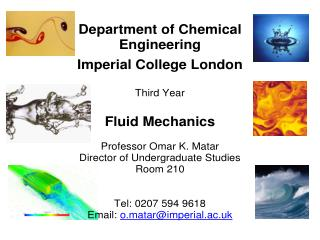DownloadDownload PresentationLecture 0: Introduction

# Lecture 0: Introduction

Download Presentation## Lecture 0: Introduction

- - - - - - - - - - - - - - - - - - - - - - - - - - - E N D - - - - - - - - - - - - - - - - - - - - - - - - - - -
##### Presentation Transcript

1. Lecture 0: Introduction Objective • To introduce the 3rd year course and look back at 2nd year Fluid Mechanics course. Contents • Course objectives • Course outline • Course materials, teaching and learning methods • Assessment methods • Pre-requisites from the 2nd year course Fluid Mechanics - OKM 2013

2. Course objectives • To introduce the concept of streamfunction, vorticity, and potential. • To solve problems in Stokes (slow) and potential (fast) flows. • To introduce concepts in two-phase flows. • To solve problems in two-phase flows. • To introduce rheological models for non-Newtonian fluids. • To solve flow problems involving non-Newtonian fluids. • To introduce the concepts of flow instability and models for turbulence. • To solve problems involving turbulent flows. Fluid Mechanics - OKM 2013

3. Course outline • Streamfunctions, vorticity, and potential (1 lecture) • Stokes and potential flow (1 lecture) • Two-phase flows (3 lectures) • Non-Newtonian fluids: Rheology (1 lecture) • Non-Newtonian flow: Example problems (1 lectures) • Turbulence: Reynolds-averaging and the ‘closure’ problem (1 lecture) • Turbulence: ‘Mixing length’ theory (1 lectures) • Turbulence: Example problems (1 lecture) Fluid Mechanics - OKM 2013

4. Course materials, Teaching and Learning Methods Course materials • Problem sheets: • available on Blackboard; problems discussed during lectures. • Notes: • can be downloaded from Blackboard. Teaching and learning methods • Lectures: • 102-hour lectures, every Friday in the Spring Term. • Private study: • Working on problem sheets. • Reading course notes and text books. • Revising for test and exams. • Tutorials: • Discussion of lectures and problem sheets run by PGs. Fluid Mechanics - OKM 2013

5. Assessment Methods Problem sheets • 7 sheets (3 of them are for a revision of FM2) with questions to review your own progress. Exam • Transfer Processes III (3 hours) – shared with Particle Engineering and Separation Processes II. Fluid Mechanics - OKM 2013

6. Textbooks No textbook is required for this course but one may be helpful. Recommend list: • Richardson (yes, that Richardson!), Fluid Mechanics, Hemisphere. • Wilkes, Fluid Mechanics for Chemical Engineers, Prentice-Hall • Denn, Process Fluid Mechanics, Prentice-Hall. • Massey, Mechanics of Fluids, van Nostrand. • Fox & McDonald, Introduction to Fluid Mechanics, Wiley. • Streeter & Wylie, Fluid Mechanics, McGraw-Hill. Available from the Library. Try to find one that helps you. Consult me should you decide to buy one. Fluid Mechanics - OKM 2013

7. Pre-requisites from the 2nd year course • Derivation of Navier-Stokes (NS) equations using vector calculus and the divergence theorem: see FM2 lecture notes. • Solution of the NS equations for unidirectional flows: see problem sheet 1. • How to make the NS dimensionless, what is Re for some example flows: see problem sheet 2. • Self-similar solutions of the NS equations, using the product and chain rule of differentiation, and separation of variables: see problem sheet 3. • See ‘Revision of FM2’ notes on BB and basic FM equations Fluid Mechanics - OKM 2013